APP Information

 Download Version 2.44 (244) Apk Size 3.56 MB App Developer Cymath Malware Check TRUSTED Install on Android 4.2.x and up App Package com.cymath.cymath.apk MD5 68d5550bccc4ae792875d3f91a33126f Rate 4.56 Website https://www.cymath.com# Cymath - Math Problem Solver App for Android and iOS

Students in math classes can often find themselves overwhelmed at the amount of work expected and the difficulty of the equations that need solving. For those students and anyone else who needs help with their math equations, Cymath offers a convenient and easy-to-use math problem solver app for both Android and iOS devices.

## How Cymath Helps to Solve Math Problems

The Cymath app is a powerful math problem solver that can easily solve a wide range of equations. Whether it be basic math equations such as addition, subtraction, multiplication, division, linear equations, quadratic equations, or calculus problems, Cymath can easily handle them all. The app also has step-by-step solutions and a detailed explanation to all equations, making it very easy to understand why a certain answer was reached.

Using the Cymath app is incredibly easy and efficient. To use the app, all that is required is to type in the math equation, or take a picture of it and Cymath will solve the problem. Cymath is perfect for both students and teachers that need help with equations in the classroom, or anyone that needs help with a math problem.

## Features of the Cymath App Include:

• Easy to use interface
• Ability to solve a wide range of equations
• Step-by-step solution and detailed explanation for every equation
• Shareable results online
• Instant math problem solver with picture option

Cymath is a great tool for any student that needs help with a math problem. The app is incredibly easy to use, and makes it easy to understand how a certain answer was reached. Cymath is perfect for both students and teachers, and perfect for anyone that needs help with a math equation.

### App Description

```With millions of Cymath.com users worldwide, the Cymath math problem solver app uses the same math engine while letting you solve problems on the go! Just enter a problem from your math homework, and let Cymath solve it for you step-by-step! We provide algebra as well as calculus help.

Topics in algebra include: equation solving, factoring, logarithms, exponents, complex numbers, quadratic equations, trigonometry, partial fraction, polynomial division, etc. Topics in calculus include: product rule, quotient rule, chain rule, u-substitution, integration by parts, integration by partial fraction, trigonometric substitution, rationalizing substitution, and much more. We provide math answers and steps for all of the above.

In short, whether you are simply stuck on your math homework, want to check your homework answers, or need specific calculus help, let our math problem solver help now!
```

### App ChangeLog

• The Cymath app now loads faster!

### More Android Apps to Consider

1.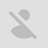HungryHumbleGrumble

I most definitely recommend this app for math. It gives the person a detailed solution and not just saying the answer. Ex: 1x4=4. It gives us an example of the problem instead of giving us what I just showed. This app deserves more than just a 4.5 rating on this app. Good work.

2.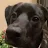Nixie

I'm so happy that I found this app, it's helped with a ton of assignments. however there's no option for distributive property, that I think would be helpful.

3.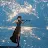I would give this 0 star if I could. What is the use of a math app without camera feature. Each time I take a photo of my question, it just tells me "No internet connection" when I connected. Then it tells me to type the question. Quite useless if you ask me. I can't even access history unless I get the pro version 👎. Uninstalled 😠

4.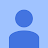Arika Moto

Just downloaded. Works excellent, so far. However, needs a Clear button so I can start a new equation without having to go back and forth between screens. Also, needs whole alphabet available (more letters than just w,x,y,z) to simplify expressions such as a²b³c-(p-q+r)=s+t

5.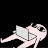Catalina Talavera

I would definitely recommend this app to other people it's easy and doesn't really need much of your personal info I'd say it's better then alot of the apps I have tried!¹

6.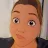Michelle Marsh

So easy to use. Really helps my kids with understanding how to do different types of math problems by showing how to do the work.

7.Mason_4135

5/5 Helped me understand math more easy and I also liked the features where you can chose to take a photo of the problem or type it and it will lead you step by step. I also liked how you can chose what type of problem it is such as simplifying,etc...

8.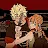August Baldwin

Helps alot in math class when I don't understand something I can just pull out my phone and type the problem up or take a photo and it'll solve it for me and my teacher doesn't even notice I use an app to cheat

9.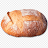Kermit

This thing is so damn good it explains how to do the problem and gives the answer plus it has no subscriptions

10.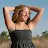Calysta Benigni

Great all so far! Highly recommend for all math. You can write the problem and them help you, or you can take a pic. So great! I hope you all love this app as much as I do!!